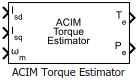Main Content

# ACIM Torque Estimator

Estimate electromechanical torque and power

• Library:
• Motor Control Blockset / Controls / Control Reference

•## Description

The ACIM Torque Estimator block generates electromechanical torque and power estimates for an induction motor. The block outputs the mathematically computed electromechanical torque for constant motor parameters. To measure an accurate torque value, we recommend that you use a physical sensor.

The block accepts feedback values of d- and q-axis stator current and mechanical speed as inputs.

### Equations

If you select `Per-Unit (PU)` in the Input units parameter, the block converts the inputs to SI units before performing any computation. After calculating the output, the block converts the output back to per unit values.

These equations describe the computation of electromechanical torque and power estimates by the block.

`${T}_{e}=\frac{3}{2}p\left(\frac{{L}_{m}}{{L}_{r}}\right){\lambda }_{rd}{i}_{sq}$`
`${P}_{\text{e}}={T}_{e}\cdot {\omega }_{\text{m}}$`

For detailed set of equations and assumptions, see Mathematical Model of Induction Motor.

where:

• $p$ is the number of pole pairs available in the motor.

• ${L}_{m}$ is the magnetizing inductance of the motor (Henry).

• ${L}_{r}$ is the rotor inductance (Henry).

• ${\lambda }_{rd}$ is the d-axis flux linkage of the rotor (Weber).

• ${i}_{sq}$ is the stator q-axis current (Amperes).

• ${\omega }_{m}$ is the mechanical speed of the rotor (Radians/ sec).

## Ports

### Input

expand all

Stator current along the d-axis of the rotating dq reference frame.

Data Types: `single` | `double` | `fixed point`

Stator current along the q-axis of the rotating dq reference frame.

Data Types: `single` | `double` | `fixed point`

Mechanical speed of the rotor.

Data Types: `single` | `double` | `fixed point`

### Output

expand all

Electromechanical torque of the rotor.

Data Types: `single` | `double` | `fixed point`

Electromechanical power of the rotor.

Data Types: `single` | `double` | `fixed point`

## Parameters

expand all

Number of pole pairs available in the induction motor.

Inductance due to leakage flux linked to the stator winding (in Henry).

Inductance due to leakage flux linked to the rotor winding (in Henry).

Inductance due to the magnetizing flux (in Henry)..

Rated flux of the induction motor (in Weber).

Unit of the input values.

Base voltage (in Volts) for per-unit system.

#### Dependencies

To enable this parameter, set Input units to `Per-Unit (PU)`.

Base current (in Amperes) for per-unit system.

#### Dependencies

To enable this parameter, set Input units to `Per-Unit (PU)`.

Base speed (in rpm) for per-unit system.

#### Dependencies

To enable this parameter, set Input units to `Per-Unit (PU)`.

Base torque (in Nm) for per-unit system. See Per-Unit System page for more details.

This parameter is not configurable and uses a value that is internally computed using other parameters.

#### Dependencies

To display this parameter, set Input units to `Per-Unit (PU)`.

Base power (in W) for per-unit system. See Per-Unit System page for more details.

This parameter is not configurable and uses a value that is internally computed using other parameters.

#### Dependencies

To display this parameter, set Input units to `Per-Unit (PU)`.

## See Also

### Topics

Introduced in R2020b

Get ebook